# 接缝雕刻算法:一种看似不可能的图像大小调整方法

2020/11/09 23:03

### 介绍

（本文的灵感来自麻省理工学院的格兰特·桑德森的演讲。）

### 问题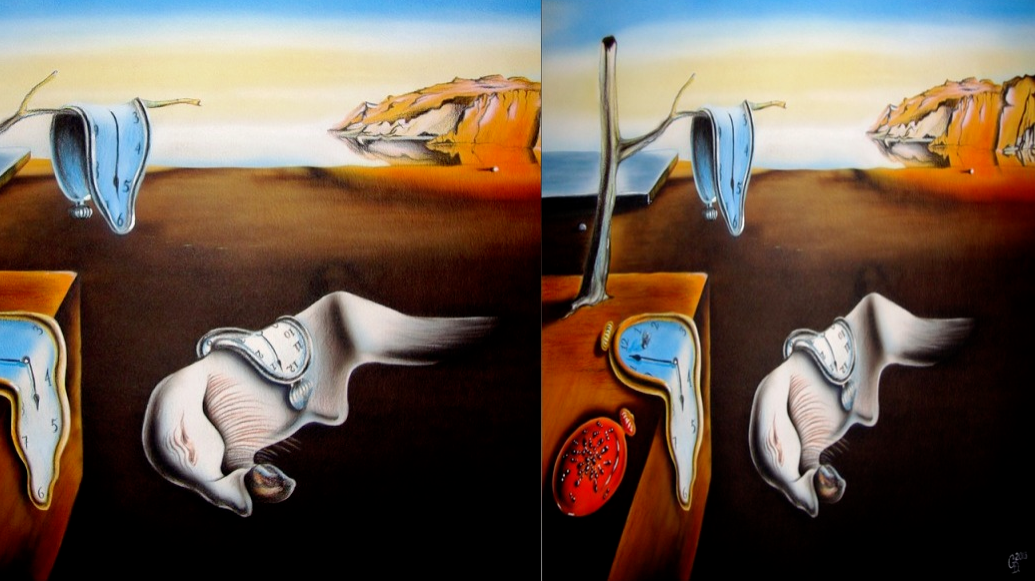1. 识别图片中有趣的部分（即对象）。

2. 标识可以去除而不会扭曲图片的像素路径。

### 识别对象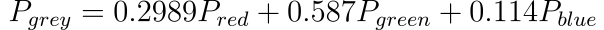def rgbToGrey(arr):
greyVal = np.dot(arr[...,:3], [0.2989, 0.5870, 0.1140])
return np.round(greyVal).astype(np.int32)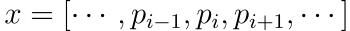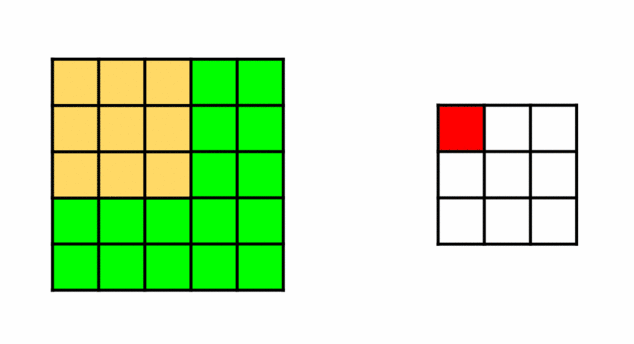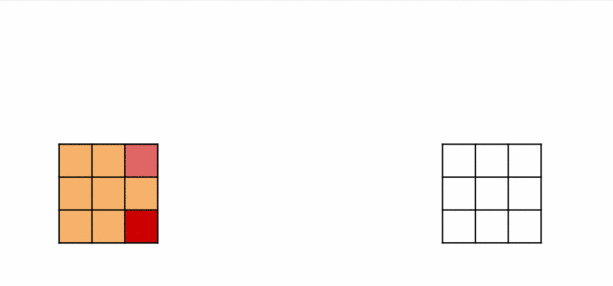def naiveConvolve(img,ker):

res = np.zeros(img.shape)
r,c = img.shape
rK,cK = ker.shape
halfHeight,halfWidth = rK//2,cK//2

ker = np.rot90(ker,2)

for i in range(1,r+1):
for j in range(1,c+1):
res[i-1,j-1] = np.sum(np.multiply(ker,img[i-halfHeight:i+halfHeight+1,j-halfWidth:j+halfWidth+1]))

return res，其中F（w）表示频域中的函数。

def fastConvolve(img,ker):
imgF = np.fft.rfft2(img)
kerF = np.fft.rfft2(ker,img.shape)
return np.fft.irfft2(imgF*kerF)


（注意：在某些情况下，得出来的值可能与朴素方法稍有不同，因为fastConvolve函数会计算圆形卷积。但是实际上，我们可以轻松地使用快速卷积，而不必担心这些较小的值差异。）def getEdge(greyImg):

sX = np.array([[0.25,0.5,0.25],
[0,0,0],
[-0.25,-0.5,-0.25]])
sY = np.array([[0.25,0,-0.25],
[0.5,0,-0.5],
[0.25,0,-0.25]])

#edgeH = naiveConvolve(greyImg,sX)
#edgeV = naiveConvolve(greyImg,sY)
edgeH = fastConvolve(greyImg,sX)
edgeV = fastConvolve(greyImg,sY)

return np.sqrt(np.square(edgeH) + np.square(edgeV))


### 识别像素路径：

1. 在最底行（即i = r-1）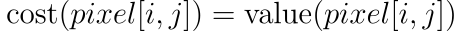1. 对于任何中间像素，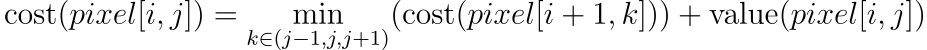def findCostArr(edgeImg):
r,c = edgeImg.shape
cost = np.zeros(edgeImg.shape)
cost[r-1,:] = edgeImg[r-1,:]

for i in range(r-2,-1,-1):

for j in range(c):
c1,c2 = max(j-1,0),min(c,j+2)
cost[i][j] = edgeImg[i][j] + cost[i+1,c1:c2].min()

return cost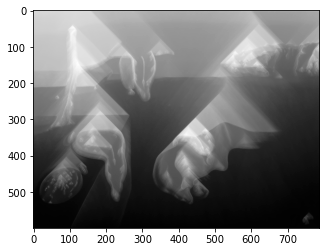def findSeam(cost):

r,c = cost.shape

path = []
j = cost.argmin()
path.append(j)

for i in range(r-1):
c1,c2 = max(j-1,0),min(c,j+2)
j = max(j-1,0)+cost[i+1,c1:c2].argmin()
path.append(j)

return path


def removeSeam(img,path):
r,c,_ = img.shape
newImg = np.zeros((r,c,3))
for i,j in enumerate(path):
newImg[i,0:j,:] = img[i,0:j,:]
newImg[i,j:c-1,:] = img[i,j+1:c,:]
return newImg[:,:-1,:].astype(np.int32)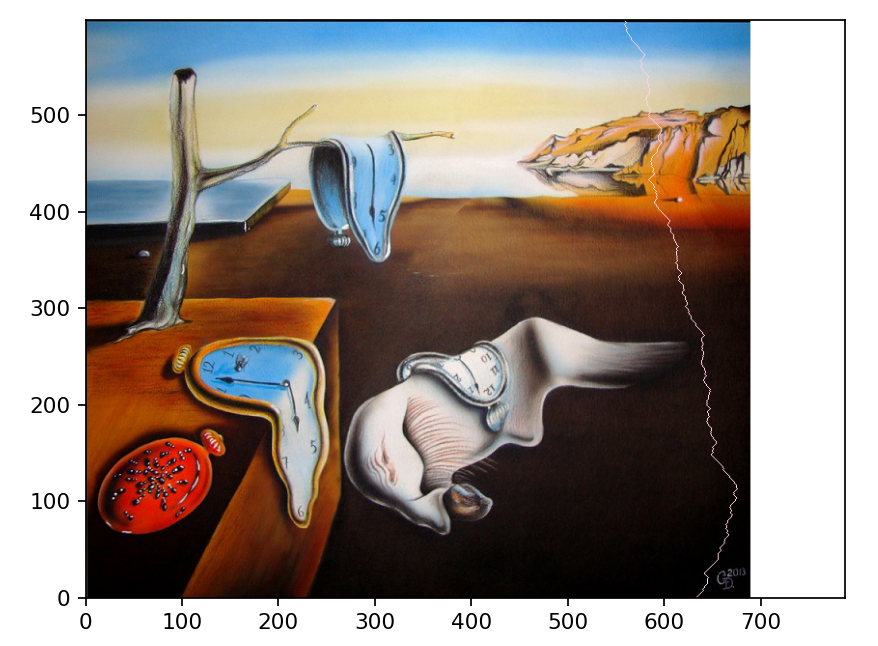sklearn机器学习中文官方文档： http://sklearn123.com/0 评论
0 收藏
0